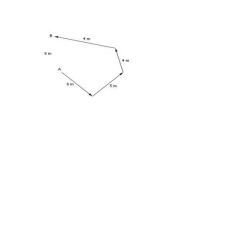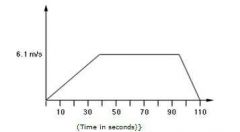• Shuffle
Toggle On
Toggle Off
• Alphabetize
Toggle On
Toggle Off
• Front First
Toggle On
Toggle Off
• Both Sides
Toggle On
Toggle Off
Toggle On
Toggle Off
Front

## Card Range To Study

throughPlay buttonPlay buttonProgress

1/10

Click to flip

Use LEFT and RIGHT arrow keys to navigate between flashcards;

Use UP and DOWN arrow keys to flip the card;

H to show hint;A body moves along a path as shown in the diagram below in a time of 3 s. What is its average velocity? A) 6.3 m/s B) 4 m/s north C) 8.3 m/s north D) 5.2 m/sE) 2 m/s north E) 2 m/s north Which of the following statements about acceleration is true? A) Time/forceB) Result of an unbalanced force exerted on a bodyC) The rate of change of displacement D) Force/time E) Time/velocity B) Result of an unbalanced force exerted on a body A body moves from Point A to Point B in a semicircular path. The diameter of the semicircle is 2 m. Calculate the distance between Point A and Point B.A) 6.28 mB) 3.14 mC) 2.07 m D) 1.04 m E) 0.52 m B) 3.14 m Velocity may be defined as:A) Speed in a given time B) SpeedC) Speed in a given direction D) Rotational speed E) Linear speed C) Speed in a given direction A vehicle that travels 150 km in 3 hours has a: A) Distance travelled of 50 kmB) Average speed of 50 km/hC) Relative velocity of 50 km/h D) Velocity of 750 km/hE) Velocity of 50 km/h B) Average speed of 50 km/hUsing the graph below, find the displacement of the object after 1 minute 50 seconds. A) 396.5 mB) 518.5 mC) 9.15 m D) 122 m E) 671 m B) 518.5 m Velocity is a/an:A) Vector quantity B) Standard quantity C) Maximum quantity D) Average quantity E) Scalar quantity A) Vector quantity Velocity may be defined as:A) Linear speed B) Speed C) Rotational speed D) Speed in a given timeE) Speed in a given direction E) Speed in a given direction Distance is: A) Always measured in a circular pathB) A scalar quantityC) Always a vector quantity D) Velocity divided by time E) Measured in a straight line B) A scalar quantity A car travels a total of 350 km. The journey involves a total of 3 hours driving time and 45 minutes of stop time. What is the average journey speed? A) 71.7 km/h B) 107.7 km/hC) 116.7 km/hD) 93.3 km/h E) 101.4 km/h D) 93.3 km/h